# [PYTHON] [Introduction to StyleGAN] I played with "The Life of a Man" ♬

To be clear, just by using Example, you can immediately reproduce the video that is often played (male ⇒ female conversion), and it is really amazing when you try it. This time, it is an introductory article that I tried only the first entrance. The reference is as follows. 【reference】 ① I tried using StyleGANTry mixing Yukichi Fukuzawa with StyleGanPuzer/stylegan-encoderNVlabs/stylegan Currently, styleGAN2 seems to be released, but I would like to take the first step of StyleGAN and get a feel for it. As a result, I was able to create the following video in almost one day, so I will write an article about what I was interested in.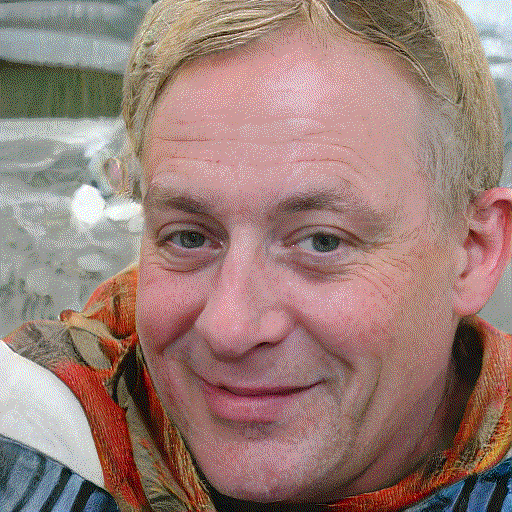• I made something that changes from old man to infant to old man, but even \$ 512 ^ 2 \$ exceeded 10MB.

### What i did

·environment ・ Run pretrained_example.py ・ Move the latent space ・ Gif animation

### ·environment

The Keras-gpu environment is broken because I installed Pytorch the other day, so I installed it from scratch. Machine; 1060 base OS；Windows10 The basis is as in the previous article. However, Chainer etc. will not be installed. When I finished building the environment, I got an error in Tensorflow. So, we have replaced it with Tensorflow = 1.13.1 as shown below. After replacing it, it doesn't work well, so I'm restarting it. 【reference】 ⑤ How to specify the build with conda install of Anaconda It worked stably with this. The evidence is as follows (the warning appears in the part of ..., but this time it has no effect, so it is deleted)

``````(keras-gpu) C:\Users\user\stylegan-encoder-master>python
Python 3.6.9 |Anaconda, Inc.| (default, Jul 30 2019, 14:00:49) [MSC v.1915 64 bit (AMD64)] on win32
>>> import tensorflow as tf
．．．
>>> print(tf.__version__)
1.13.1
``````

### ・ Run pretrained_example.py

To make this work, Weights could not be downloaded in the code as in Reference ①. Therefore, I downloaded it from Reference ⑦ below and placed it in the same way as Reference ① in the Tensorflow version. Reference ⑥ seems to be interesting, but it is still difficult to understand, so I will postpone it next time. 【reference】 ⑥ Play StyleGAN !! ~ Image editing without additional learning ~pacifinapacific/StyleGAN_LatentEditor

So the first code is:

#### `pretrained_example.py`

``````
#
# 4.0 International License. To view a copy of this license, visit
# http://creativecommons.org/licenses/by-nc/4.0/ or send a letter to
# Creative Commons, PO Box 1866, Mountain View, CA 94042, USA.

"""Minimal script for generating an image using pre-trained StyleGAN generator."""

import os
import pickle
import numpy as np
import PIL.Image
import dnnlib
import dnnlib.tflib as tflib
import config

def main():
# Initialize TensorFlow.
tflib.init_tf()
# Load pre-trained network.
#url = 'https://drive.google.com/uc?id=1MEGjdvVpUsu1jB4zrXZN7Y4kBBOzizDQ' # karras2019stylegan-ffhq-1024x1024.pkl
#with dnnlib.util.open_url(url, cache_dir=config.cache_dir) as f:
#    _G, _D, Gs = pickle.load(f)
fpath = './weight_files/tensorflow/karras2019stylegan-ffhq-1024x1024.pkl'
with open(fpath, mode='rb') as f:
_G, _D, Gs = pickle.load(f)
# _G = Instantaneous snapshot of the generator. Mainly useful for resuming a previous training run.
# _D = Instantaneous snapshot of the discriminator. Mainly useful for resuming a previous training run.
# Gs = Long-term average of the generator. Yields higher-quality results than the instantaneous snapshot.
# Print network details.
Gs.print_layers()
# Pick latent vector.
rnd = np.random.RandomState(5)
latents = rnd.randn(1, Gs.input_shape)
# Generate image.
fmt = dict(func=tflib.convert_images_to_uint8, nchw_to_nhwc=True)
images = Gs.run(latents, None, truncation_psi=0.7, randomize_noise=True, output_transform=fmt)
# Save image.
os.makedirs(config.result_dir, exist_ok=True)
png_filename = os.path.join(config.result_dir, 'example.png')
PIL.Image.fromarray(images, 'RGB').save(png_filename)

if __name__ == "__main__":
main()
``````

The essence of this code is to give a random number of the same size as the initial input value of Generator Gs below. `latents = rnd.randn(1, Gs.input_shape)` It is the part that is converted to an image below using it. `images = Gs.run(latents, None, truncation_psi=0.7, randomize_noise=True, output_transform=fmt)` 【reference】 ⑧ Random number generation summary by Numpy The following output with this code.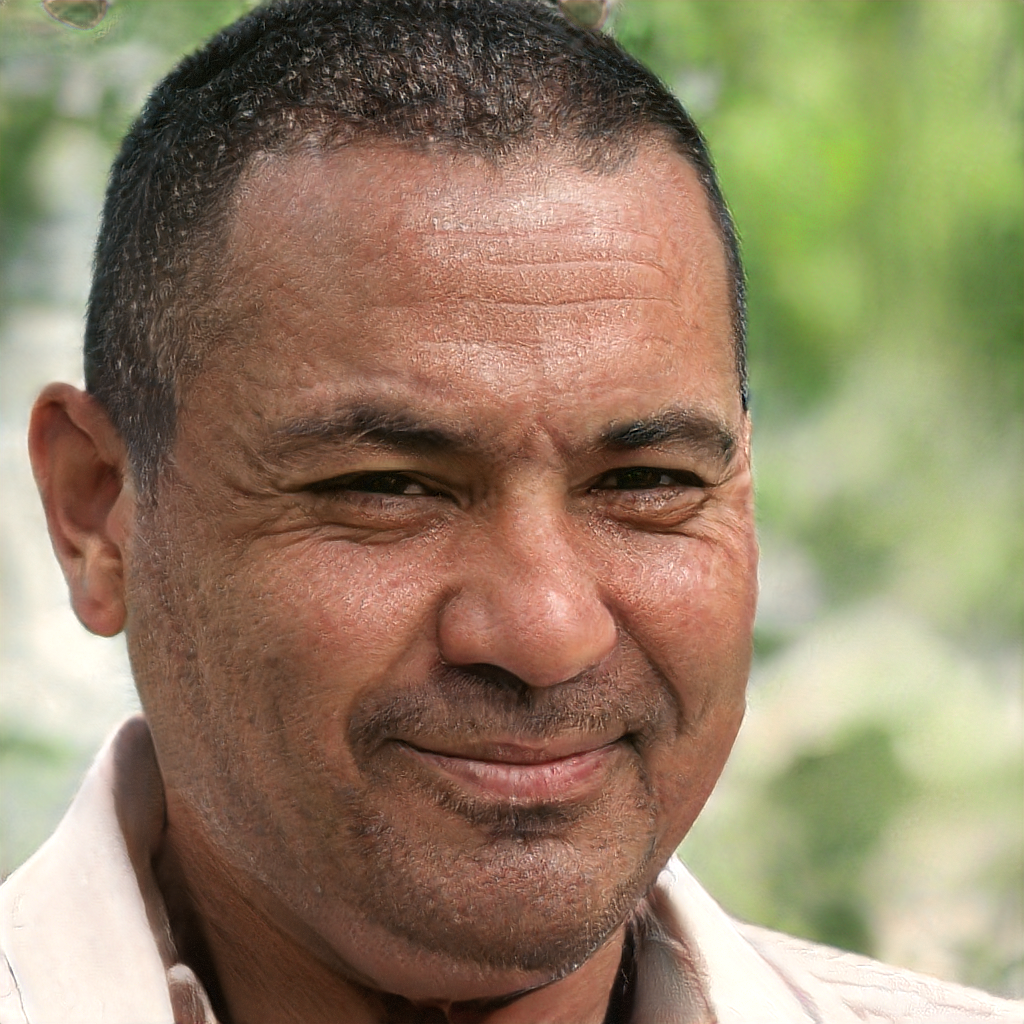### ・ Move the latent space

The next thing to do is to change the random numbers in the above latents and output them. So, I tried it with the following code. (If only the main part is described)

``````def main():
# Initialize TensorFlow.
tflib.init_tf()
fpath = './weight_files/tensorflow/karras2019stylegan-ffhq-1024x1024.pkl'
with open(fpath, mode='rb') as f:
_G, _D, Gs = pickle.load(f)
# Print network details.
Gs.print_layers()

# Pick latent vector.
rnd = np.random.RandomState(5) #5
latents1 = rnd.randn(1, Gs.input_shape)
latents2 = rnd.randn(1, Gs.input_shape)
for i in range(1,101,4):
latents = i/100*latents1+(1-i/100)*latents2
# Generate image.
fmt = dict(func=tflib.convert_images_to_uint8, nchw_to_nhwc=True)
images = Gs.run(latents, None, truncation_psi=0.7, randomize_noise=True, output_transform=fmt)
# Save image.
os.makedirs(config.result_dir, exist_ok=True)
png_filename = os.path.join(config.result_dir, 'example{}.png'.format(i))
PIL.Image.fromarray(images, 'RGB').save(png_filename)
``````

In other words, it is a random number, but continuous latents1 latents2 is calculated, and the parameters are changed between them to draw. With this operation, you can see how the female changes smoothly to male 1 and male 2.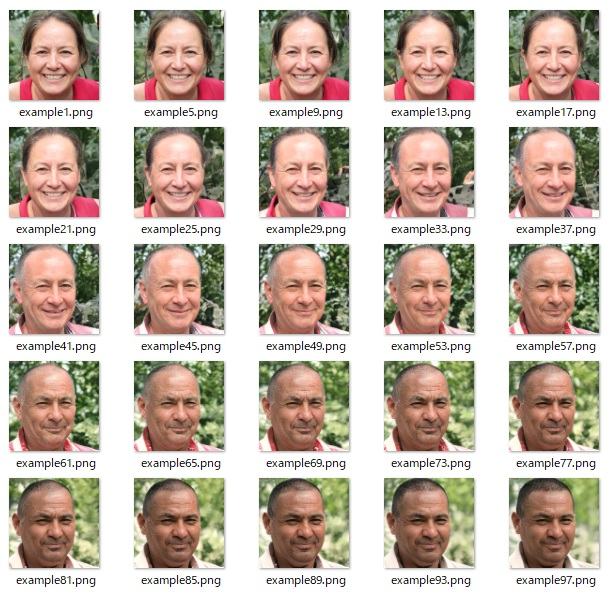Here, I thought about how to wander the latent space one step further. That is, replace that part with the following code. Now you can see what kind of image is hidden though it is part of the latent space.

``````    # Pick latent vector.
rnd = np.random.RandomState(6) #5
for i in range(1,101,4):
latents = rnd.randn(1, Gs.input_shape)
``````

I got the following: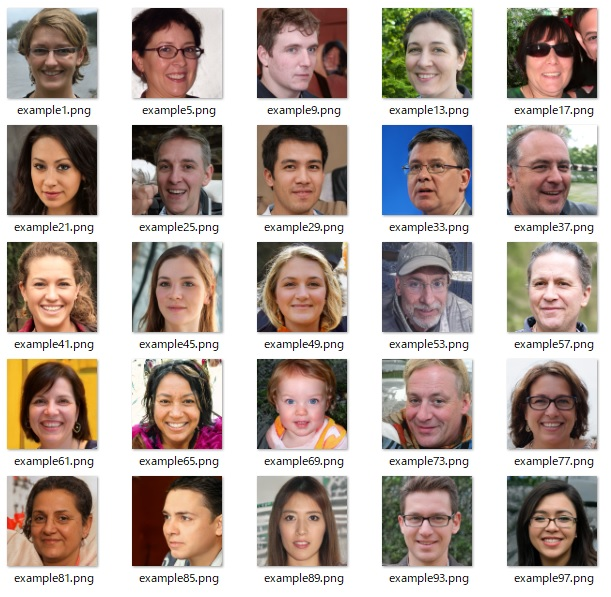When this happens, you will want to select any person in this image and see the transition. So, I tried to draw a picture of a man of example73 and a child of example69, which seems to change the most. It happens to be next to each other ... The code is below.

``````    # Pick latent vector.
rnd = np.random.RandomState(6) #5
latents_=[]
a=17
b=18
for i in range(1,101,4):
latents = rnd.randn(1, Gs.input_shape)
latents_.append(latents)

for j in range(25):
latents_mean=j/25*latents_[a]+(1-j/25)*latents_[b]
# Generate image.
fmt = dict(func=tflib.convert_images_to_uint8, nchw_to_nhwc=True)
images = Gs.run(latents_mean, None, truncation_psi=0.7, randomize_noise=True, output_transform=fmt)
# Save image.
os.makedirs(config.result_dir, exist_ok=True)
png_filename = os.path.join(config.result_dir, 'example{}.png'.format(j))
PIL.Image.fromarray(images, 'RGB').save(png_filename)
``````

The output result exceeded expectations as shown below.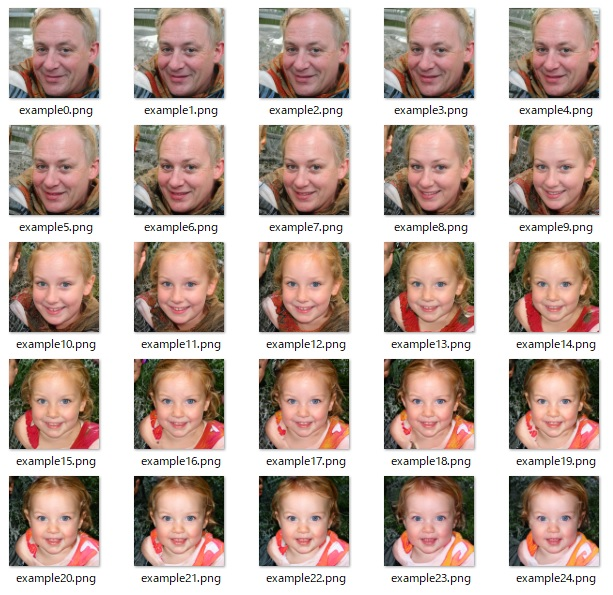It's strange that there is a picture of a child really growing up as an uncle.

### ・ Gif animation

Finally, I want to make a gif animation like everyone is doing. To do this, put the following code at the end and the gif will be saved.

• Here, I try to go back to the beginning by turning it backwards from the beginning to the end.
``````    s=50
images = []
for i in range(25):
im = Image.open(config.result_dir+'/example'+str(i)+'.png')
im =im.resize(size=(512, 512), resample=Image.NEAREST)
images.append(im)
for i in range(24,0,-1):
im = Image.open(config.result_dir+'/example'+str(i)+'.png')
im =im.resize(size=(512, 512), resample=Image.NEAREST)
images.append(im)

images.save(config.result_dir+'/example{}_{}.gif'.format(a,b), save_all=True, append_images=images[1:s], duration=100*2, loop=0)
``````

The following result is changed to size = (256, 256) due to the publication size. Also, the output is s = 25, excluding the binary interpolation of 6.

• The Gif animation at the beginning is \$ 512 ^ 2 \$. In fact, if it is \$ 1024 ^ 2 \$, a really beautiful picture will be output and it will be amazing.
random numberseed Bivalent interpolation Subspace
6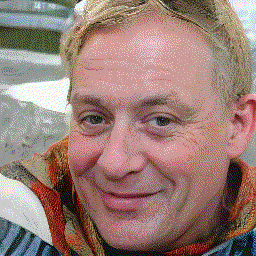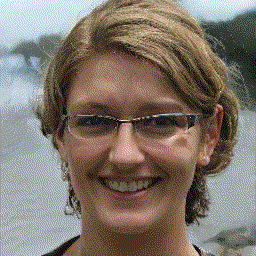5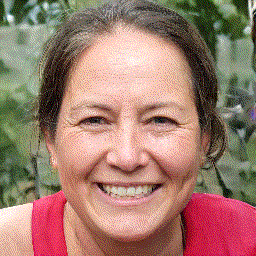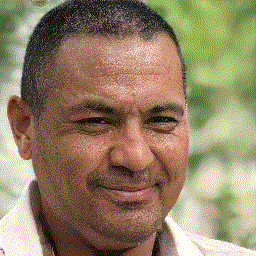### Summary

・ I think it can be moved if the environment can be constructed. ・ Great image interpolation

・ There are many possible applications for this app alone, so I'll try using my own images. ・ I would like to take on the challenge of so-called video in a more developmental way.